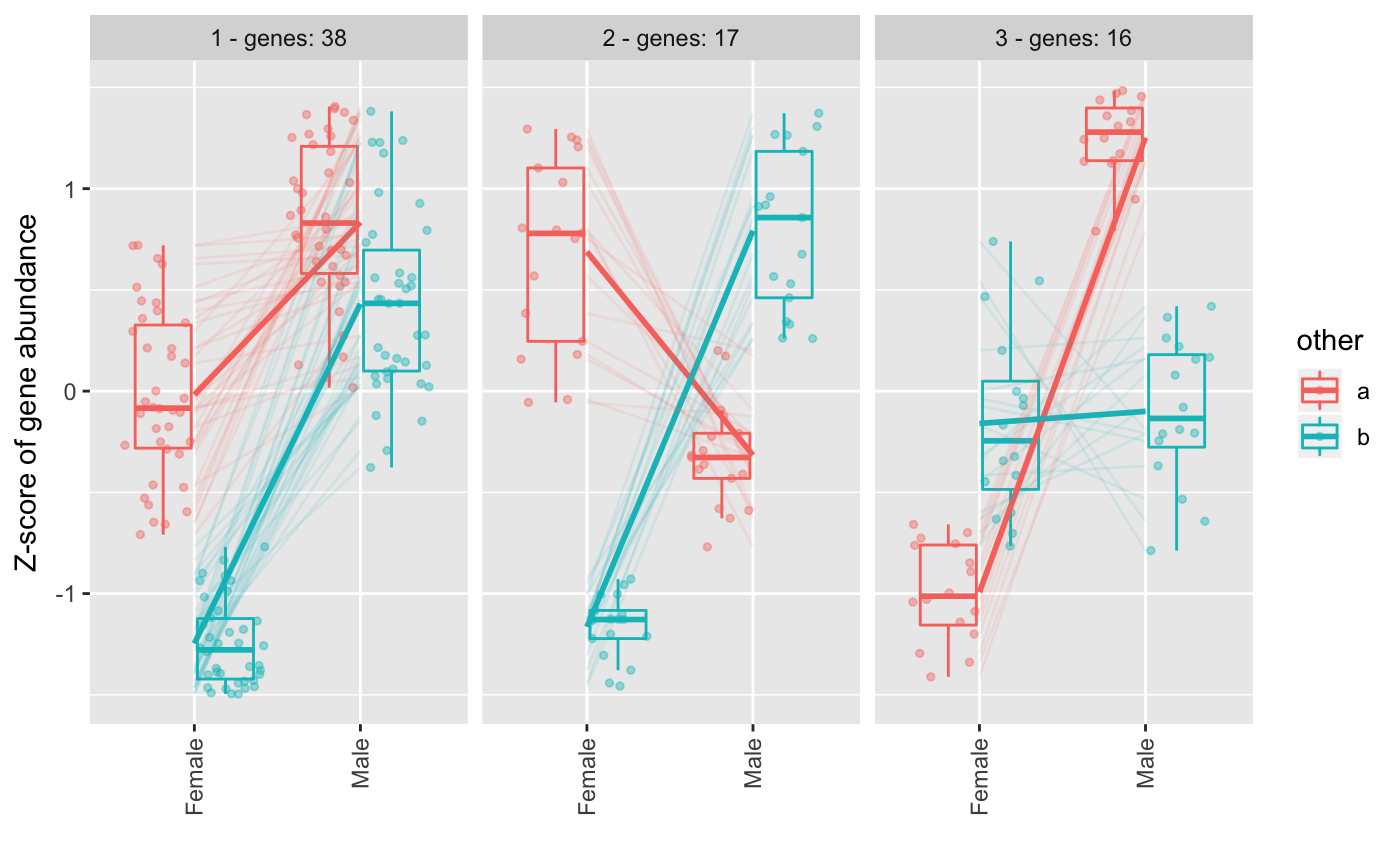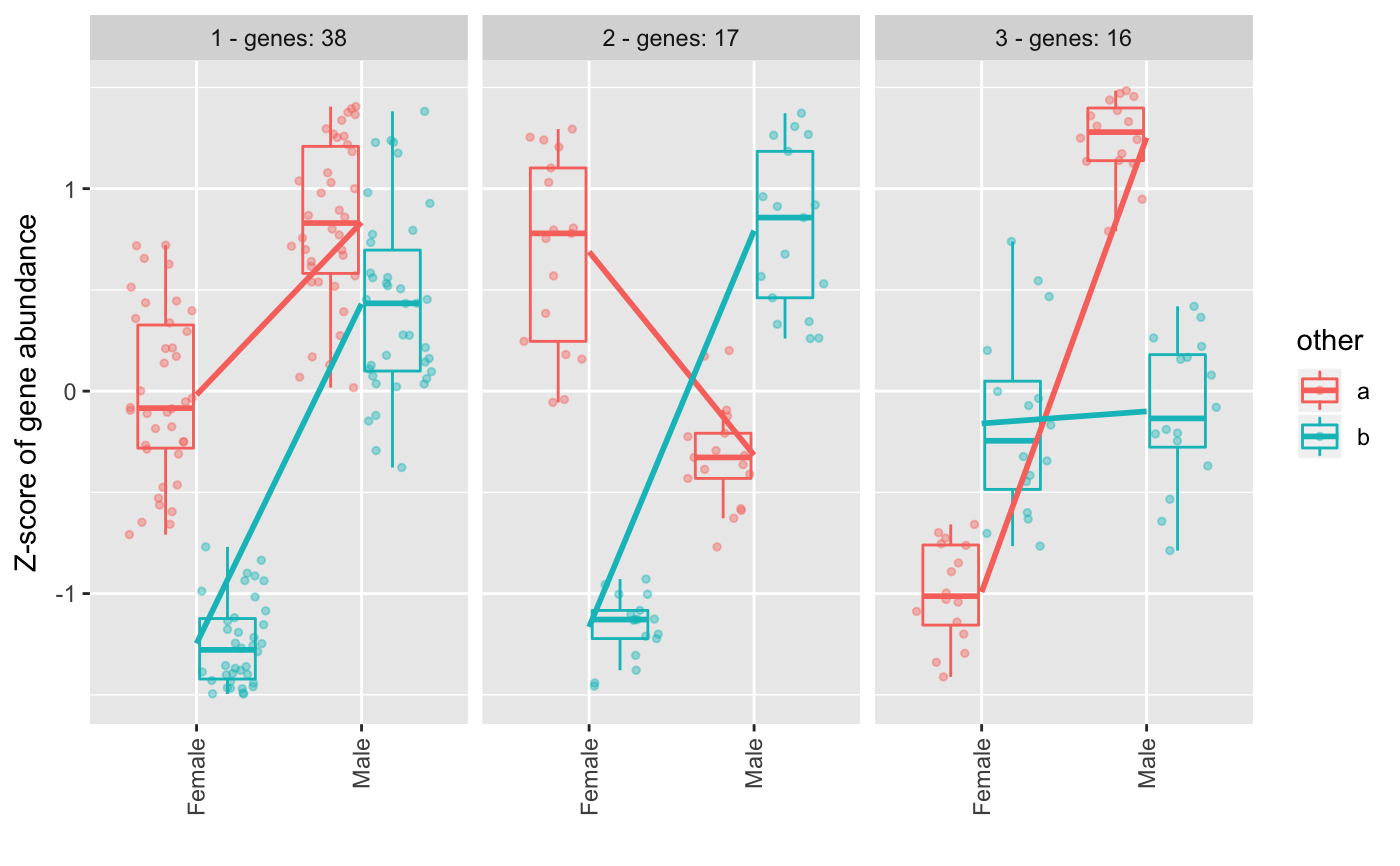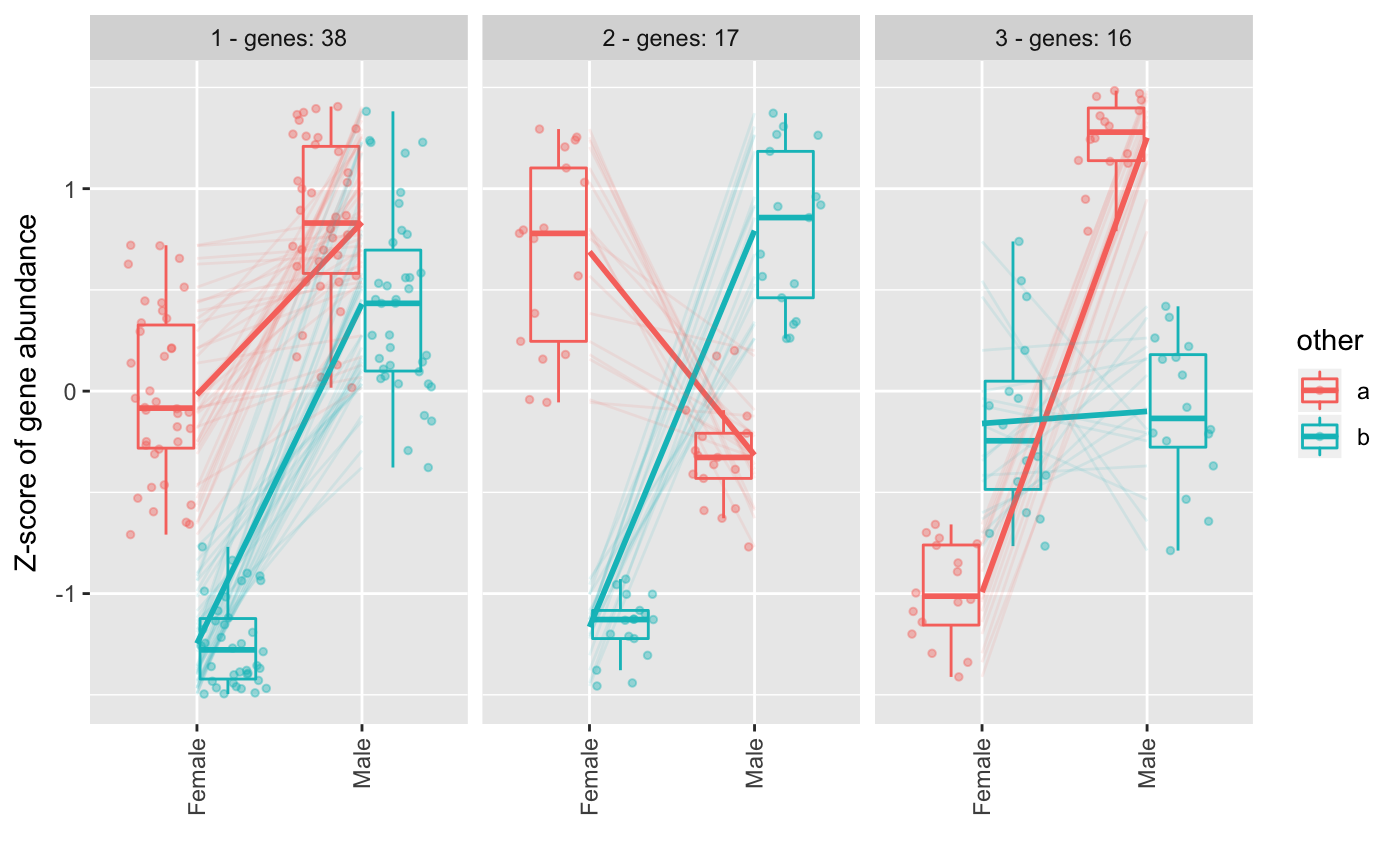This function helps to format the cluster plots from degPatterns(). It allows to control the layers and it returns a ggplot object that can accept more ggplot functions to allow customization.

degPlotCluster(table, time, color = NULL, min_genes = 10,
process = FALSE, points = TRUE, boxes = TRUE, smooth = TRUE,
lines = TRUE, facet = TRUE, cluster_column = "cluster")

## Arguments

table normalized element from degPatterns() output. It can be a data.frame with the following columns in there: genes, sample, expression, cluster, xaxis_column, color_column. column name to use in the x-axis. column name to use to color and divide the samples. whether to process the table if it is not ready for plotting. Add points to the plot. Add boxplot to the plot. Add regression line to the plot. Add gene lines to the plot. Split figures based on cluster ID. column name if cluster is in a column with a different name. Usefull, to plot cluster with different cutoffs used when grouping genes from the clustering step.

ggplot2 object.

## Examples

data(humanGender)
library(SummarizedExperiment)
library(ggplot2)
ma <- assays(humanGender)[][1:100,]
des <- colData(humanGender)
des[["other"]] <- sample(c("a", "b"), 85, replace = TRUE)
res <- degPatterns(ma, des, time="group", col = "other", plot = FALSE)#> Working with 100 genes.#> Working with 71 genes after filtering: minc > 15#> Joining, by = "merge"#> Joining, by = "merge"degPlotCluster(res$normalized, "group", "other")degPlotCluster(res$normalized, "group", "other", lines = FALSE)library(dplyr)
library(tidyr)#>
#> Attaching package: ‘tidyr’#> The following object is masked from ‘package:S4Vectors’:
#>
#>     expandlibrary(tibble)
table <- rownames_to_column(as.data.frame(ma), "genes") %>%
gather("sample", "expression", -genes) %>%
right_join(distinct(res\$df[,c("genes", "cluster")]),
by = "genes") %>%
left_join(rownames_to_column(as.data.frame(des), "sample"),
by = "sample") %>%
as.data.frame()
degPlotCluster(table, "group", "other", process = TRUE)